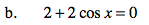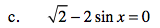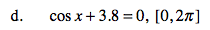### Home > PC > Chapter 6 > Lesson 6.1.4 > Problem6-56

6-56.
1. Solve for x on the indicated domain. Begin by solving for the function. Recall that if no domain is indicated, the domain is all real numbers. Remember to use either a unit circle or wave graph as a visual reminder. Homework Help ✎

1. 2 sin x − 1 = 0

2. 2 + 2 cos x = 0

3.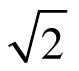− 2 sin x = 0

4. cos x + 3.8 = 0, [0, 2π]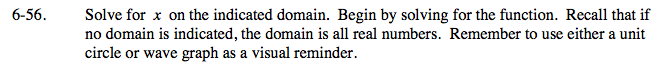Use the hints provided in the question.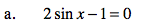n is an integer